# Class 12 Maths NCERT Solutions for Chapter 4 Determinants Exercise 4.3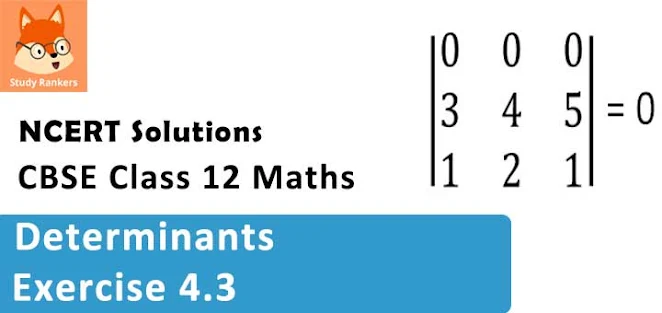### Determinants Exercise 4.3 Solutions

1. Find area of the triangle with vertices at the point given in each of the following :

(i) (1, 0) , (6, 0) , (4, 3)
(ii) (2, 7), (1, 1) , (10 , 8)
(iii) (-2, -3), (3, 2) , (-1, -8)

Solution

(i) The area of the triangle with vertices (1, 0),  (6, 0), (4, 3) is given by the relation,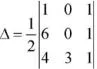= (1/2) [1(0 - 3) - 0(6 - 4) + 1(18 - 0)]
= (1/2)[-3 + 18]
= (1/2) 
= 15/2
Hence, area of the triangle is 15/2 square units.

(ii) The area of the triangle with vertices (2, 7), (1, 1), (10, 8) is given by the relation,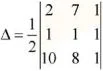= (1/2)[2(1-8) -7(1-10) +1(8-10)]
= (1/2)[ -14 + 63 - 2]
= (1/2)
= 47/2
Hence, area of the triangle is 47/2 square units.

(iii) The area of the triangle with vertices (-2, -3) , (3, 2), (-1, -8) is given by the relation,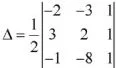= (1/2)[-2(2 + 8) + 3(3 + 1) + 1(-24 + 2)]
= (1/2)[-2(10) + 3(4) + 1(-22)]
= (1/2)[-20 + 12 - 22]
= (1/2) [-30]
= -30/2 = -15
Hence, area of the triangle is 15 square units.

2. Show that the points A(a, b + c), B(b, c + a), C(c, a +  b) are collinear.

Solution

The area of the triangle with vertices A(a, b + c), B(b, c + a), C(c, a +  b) is given by the absolute value of the relation :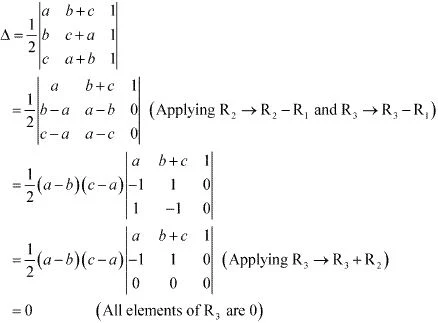Thus, the area of the triangle formed by points A, B, and C is zero.
Hence, the points A, B and C are collinear.

3. Find values of k if area of triangle is 4 square units and vertices are :
(i) (k, 0),(4, 0), (0, 2)
(ii) (-2, 0), (0, 4), (0, k)

Solution

We know that the area of a triangle whose vertices are (x1 , y1), (x2 , y­2) and (x3 , y3 ) is the absolute value of the determinant (Δ), where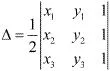If is given that the area of triangle is 4 square units.
∴ Δ＝± 4.

(i) The are of the triangle with vertices (k, 0), (4, 0) , (0, 2) is given by the relation,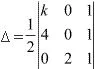= (1/2)[k(0 - 2) - 0(4 - 0) + 1(8 - 0)]
= (1/2) [-2k + 8] = -k + 4
∴  - k + 4 = ± 4
When -k + 4 = -4 , k = 8
When -k + 4 = 4 , k = 0
Hence, k = 0, 8

(ii) The are of the triangle with vertices (-2, 0), (0, 4), (0, k) is given by the relation,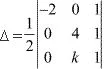= (1/2) [-2(4 - k)
= k - 4
∴  k - 4 = ± 4
When k - 4 = - 4 , k = 0
When k - 4 = 4, k = 8
Hence, k = 0, 8

4. (i) Find equation of line joining (1, 2) and (3, 6) using determinants.
(ii) Find equation of line joining (3, 1) and (9, 3) using determinants.

Solution

(i) Let P(x, y) be any point on the line joining points A(1, 2) and B(3, 6). Then, the points A, B, and P are collinear. Therefore, the area of triangle ABP will be zero.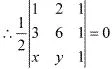⇒ 1/2 [ 1(6 - y) - 2(3 - x) + 1(3y - 6x)] = 0
⇒ 6 - y - 6 + 2x + 3y - 6x = 0
⇒ 2y - 4x = 0
⇒ y = 2x
Hence, the equation of the line joining the given points is y = 2x.

(ii) Let P (x, y) be any point on the line joining points A(3, 1) and B(9, 3). Then, the points A, B, and P are collinear. Therefore, the area of triangle ABP will be zero.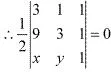⇒ 1/2 [3(3 - y) - 1(9 - x) + 1(9y - 3x)] = 0
⇒ 9 - 3y - 9 + x + 9y - 3x = 0
⇒ 6y - 2x = 0
⇒ x - 3y = 0
Hence, the equation of the line joining the given points is x - 3y = 0.

5. If area of triangle is 35 square units with vertices (2, −6), (5, 4), and (k, 4). Then k is
(A) 12
(B) −2
(C) −12, −2
(D) 12, −2

Solution

The area of the triangle with vertices (2, -6), (5, 4), and (k, 4) is given by the relation ,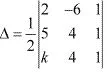= 1/2 [2(4 - 4) + 6(5 - k) + 1(20 -4k)]
= 1/2 [30 - 6k + 20 - 4k ]
= 1/2 [50 - 10k]
= 25 - 5k
It is given that the area of the triangle is ± 35.
Therefore, we have :
⇒ 25 - 5k = ± 35
⇒ 5(5 - k) = ± 35
⇒ 5 - k = ± 7
When 5 - k = - 7 , k = 5 + 7 = 12
When 5 - k = 7 , k = 5 - 7 = - 2
Hence, k = 12. -2.